# How to Calculate the Length of Service from Join Date to Current Date in Excel?

In previous article, we have learned how to calculate the hire date from birth date in excel, this time we learn something else. Suppose we have a company and several employees, and we want to count the length of service for each employee from their join date to current date, then we will know their years of work experience in our company. So, we need to know the formula to calculate the ‘Duration’. This article aims at to tell you how to calculate length of service by a simple formula, please read it and I’m sure you can get what you want.

To demonstrate the formula clearly, we create a table to show the details.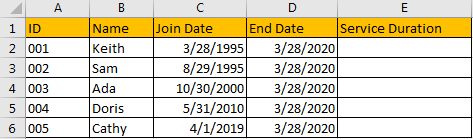Suppose we have a company with only above five employees and their join dates are different. We want to calculate the ‘Service Duration’ comparing to current date for everyone, actually we need to know the period between ‘Join Date’ and ‘End Date’.

## Part 1: Calculate Length of Service to Year & Month Unit in Excel

Step 1: In E2, enter the formula =DATEDIF(C2,D2,”y”)&” years “&DATEDIF(C2,D2,”ym”)&” months”.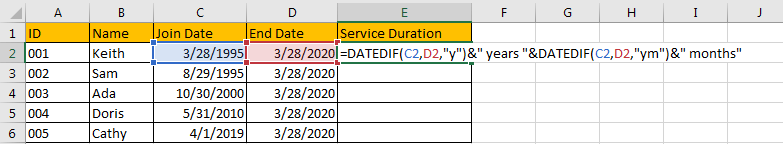1. DATEIF function returns the duration from two dates, it has three parameters like DATEDIF(start_date,end_date,unit).
2. In this case, for the first DATEIF function, the unit is ‘y’, so it returns Year duration between start date and end date; for the second DATEIF function, the unit is ‘ym’, it returns the Month duration between start date and end date (ignore Year part).
3. We use & to combine DATEIF function returned values and string “years” and “months” to make result looks reasonable.

Step 2: Click Enter to get result. See, the length of service for Keith is 25 years 0 months. This result looks very clearly.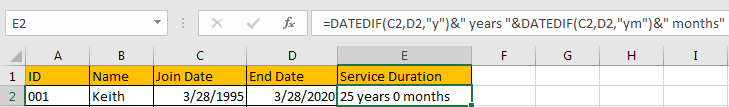Step 3: Drag the fill handle to fill cells for other employees in this table.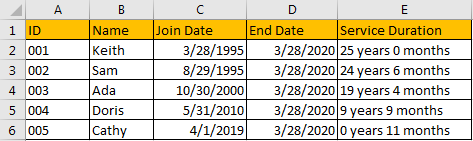## Part 2: Calculate Length of Service to Year & Month & Date Unit in Excel

You can see that in above table, though we can get a rough value about the duration, if we want to get the exact length of service, like calculate the duration end to Date unit, you can change your formula correspondingly.

Step 1: In E2, enter the formula =DATEDIF(C2,D2,”y”)&” years “&DATEDIF(C2,D2,”ym”) &” months “&DATEDIF(C2,D2,”md”) &” dates”. This time, we add date in the formula, then we can get an exact value of service duration. Make sure that for the third DATEIF function, the unit is ‘md’, otherwise the date is calculated improperly.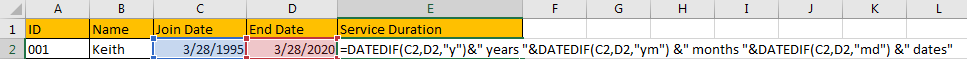Step 2: Click Enter to get result. Verify that ‘X dates’ is added in duration this time. We can repeat above step#3 to fill the other cells properly.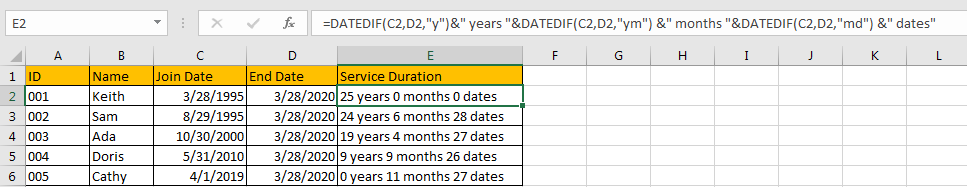Comment:

If you enter the formula like =DATEDIF(C2,D2,”y”)&” years “&DATEDIF(C2,D2,”ym”) &” months “&DATEDIF(C2,D2,”d”) &” dates”, you will get below result improperly. So, you must enter correct unit ‘md’ in formula.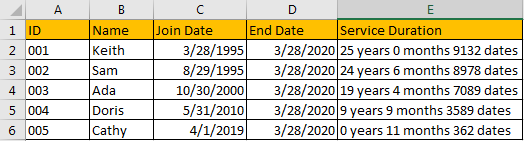## Part 3: Calculate Length of Service to Year/Month/Date Unit in Excel

Step 1: Only calculate the length of service with Year unit. Enter the formula =DATEDIF(C2,D2,”y”)&” years “.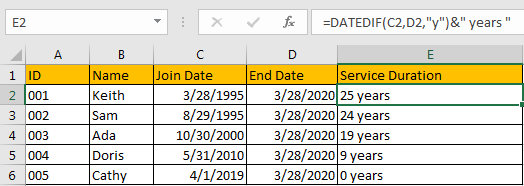Step 2: Only calculate the length of service with Month unit. Enter the formula =DATEDIF(C2,D2,”m”)&” months “.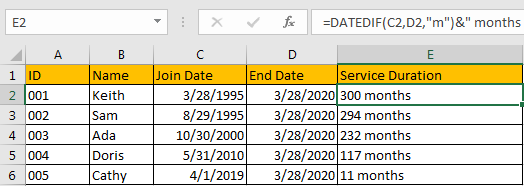Step 3: Only calculate the length of service with Date unit. Enter the formula =DATEDIF(C2,D2,”d”)&” dates “.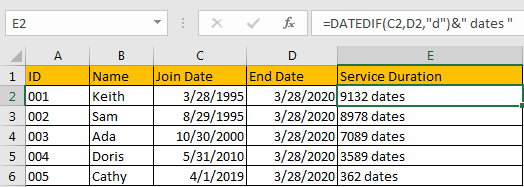Now you already know the ways to calculate service of length by different units. You can also edit your own formula in your work to suit your demands accordingly.

Related Posts

Calculate Number of Hours between Two Times

Calculating the difference between two times might be a valuable statistic for subsequent computations or averages, whether you're producing a time sheet for staff or recording personal exercises. While Excel has a plethora of complex functions, including date and time ...

Calculate Loan Interest in Given Year

When you borrow money, you are supposed to repay it gradually. Lenders, on the other hand, want to be compensated for their services and the risk they incur by lending you money. That is, you will not just repay the ...

Calculate Interest Rate for Loan

The interest rate is the fee charged by a lender to a borrower and is expressed as a percentage of the principal—the lent amount. The interest rate on a loan is often expressed as an annual percentage rate, abbreviated as ...

Calculate Interest for Given Period

Using the IPMT function in Excel, we can compute the interest payment on any loan. This step-by-step tutorial will guide Excel users of all skill levels through the process to calculate interest for given period. Finally, the formula: =IPMT(B3/12,1,B5,-B2) The ...

How To Use Excel GCD Function

This post will guide you how to use Excel GCD function with syntax and examples in Microsoft excel. Description The Excel GCD function Returns the greatest common divisor of two or more integers. So you can use the GCD function ...

Calculate A Ratio From Two Numbers In Excel

In elementary mathematics, a ratio is a connection or comparison between two or more integers. For example, ratios are often expressed as ":" to demonstrate the relationship between two numbers. You would think that manually calculating a ratio from two ...

How To Use Excel RRI Function

This post will guide you how to use Excel RRI function with syntax and examples in Microsoft excel. Description The Excel RRI function Returns an equivalent interest rate for the growth of an investment. So you can use the RRI ...

CAGR Formula Examples in Excel

CAGR in Excel is a formula that calculates the compound annual growth rate for any invested amount over the specified years or timeframe. Although there is no direct function in Excel that can help us identify the CAGR value, there ...

Build Hyperlink With VLOOKUP in Excel

You might have come across a task in which you were assigned to build hyperlinks, which seems very easy, and if you are new to excel or don't have enough experience with it, then you might wonder about doing this ...

Break ties with helper COUNTIF and column

Suppose you got a task to adjust the values that contain the ties; what would be your first attempt to break the ties of the given value? If you are wondering about doing this task manually, let me add that ...

Sidebar